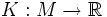# Gauss-Kronecker curvature of a hypersurface

## Definition

Let$M$ be a hypersurface in$\R^{n+1}$: in other words$M$ is a$n$-dimensional manifold embedded inside$\R^{n+1}$. The Gauss-Kronecker curvature of$M$ is a function:$K:M \to \R$

defined in the following equivalent ways:

•$K$ is the Jacobian determinant of the Gauss map of$M$
•$K$ is the determinant of the shape operator for$M$

## Particular cases

When$n = 2$, we get the usual notion of Gaussian curvature.